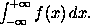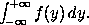### Defining your own Control Sequences in LaTeX

Suppose that we are producing a paper that makes frequent use of some mathematical expression. For example, suppose that integrals likeoccur frequently throughout the text. This formula is obtained by typing
$\int_{-\infty}^{+\infty} f(x)\,dx.$

It would be nice if we could type \inftyint (say) to obtain the integral sign at the beginning. This can be done using \newcommand. What we do is to place a line with the command
\newcommand{\inftyint}{\int_{-\infty}^{+\infty}}

near the beginning of the input file (e.g., after the \documentclass command but before the \begin{document} command). Then we only have to type
$\inftyint f(x)\,dx.$

to obtain the above formula.

We can modify this procedure slightly. Suppose that we we defined a new control sequence \intwrtx by putting the line

\newcommand{\intwrtx}{\int_{-\infty}^{+\infty} #1 \,dx}

at the beginning of the input file. If we then type the line
$\intwrtx{f(x)}.$

then we obtainWhat has happened is that the expression in braces after \intwrtx has been substituted in the expression defining \intwrtx, replacing the #1 in that expression.

The number 1 inside square brackets in the \newcommand line defining \intwrtx indicates to LaTeX that it is to expect one expression (in braces) after \intwrtx to substitute for #1 in the definition of \intwrtx. If we defined a control sequence \intwrt by

\newcommand{\intwrt}{\int_{-\infty}^{+\infty} #2 \,d #1}

then it would expect two expressions to substitute in for #1 and #2 in the definition of \intwrt. Thus if we then type
$\intwrt{y}{f(y)}.$

we obtain